## Day 2, Problem 1 - Applications of Arctangent

The inverse tangent function can be expressed as an infinite series, as shown below:

(1)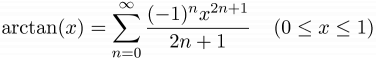$\displaystyle \arctan(x) = \sum_{n=0}^{\infty}\frac{(-1)^{n}x^{2n+1}}{2n+1}\;\;\;\;(0\le x\le 1)$

It is commonly known that the inverse tangent function can be used to compute π. For example, an easy way to compute π is using the method:

(2)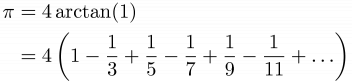\begin{align*}\pi &= 4\arctan(1)\\&=4\left ( 1-\frac{1}{3}+\frac{1}{5}-\frac{1}{7}+\frac{1}{9}-\frac{1}{11}+\ldots \right ) \end{align*}

Of course, this method is rather inefficient. We can apply the tangent angle sum identity:

(3)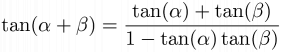$\displaystyle \tan(\alpha+\beta)=\frac{\tan(\alpha)+\tan(\beta)}{1-\tan(\alpha)\tan(\beta)}$

After some simple manipulation, the follow is obtained:

(4)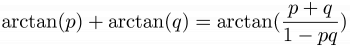$\displaystyle \arctan(p) + \arctan(q) = \arctan(\frac{p+q}{1-pq})$

Using this identity, let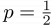$\textstyle p=\frac{1}{2}$ and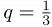$\textstyle q=\frac{1}{3}$, then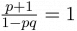$\textstyle \frac{p+1}{1-pq}=1$. Therefore: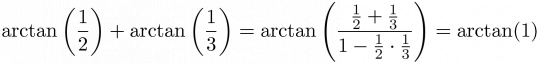$\displaystyle \arctan\left(\frac{1}{2}\right)+\arctan\left(\frac{1}{3}\right)=\arctan\left(\frac{\frac{1}{2}+\frac{1}{3}}{1-\frac{1}{2}\cdot \frac{1}{3}}\right)=\arctan(1)$

Using the inverse tangents of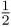$\textstyle \frac{1}{2}$ and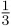$\textstyle \frac{1}{3}$ to calculate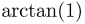$\textstyle \arctan(1)$, the speed is drastically improved.

We take equation (4) and write it in the following form: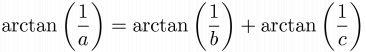$\displaystyle \arctan\left(\frac{1}{a}\right) = \arctan\left(\frac{1}{b}\right)+\arctan\left(\frac{1}{c}\right)$

where a, b, and c are each positive integers.

The problem is, for a given a (1 ≤ a ≤ 60000), find the value of b + c. It is guaranteed that for any a there will always exist an integer solution. If there are multiple solutions, you are required to find the minimum value of b + c.

### Input Format

The input consists of a single positive integer a, where 1 ≤ a ≤ 60000.

### Output Format

The output should contain a single integer, the value of b + c.

### Sample Input

1

### Sample Output

5

Point Value: 15
Time Limit: 0.25s
Memory Limit: 64M